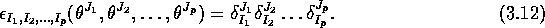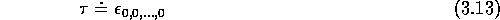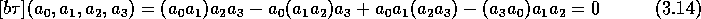Next: The dimension of the Up: Hochschild and cyclic cohomologies Previous: Closed currentsdivergence-free Grassmann-

## The hierarchy of cocycles

Let be a chart in Gr(N) and denote the corresponding lexicographic basis. We will denote by the dual basis of , i.e. . Clearly the direct product is spanned by whereIn section 3 we already called the fundamental character of Gr(N), i.e. assigns to each element of the Grassmann algebra its ``scalar'' part. We keep the same notation for>From its definition, we see that, considered as a multilinear form on Gr(N), vanishes on homogeneous elements unless all elements are -numbers. In this case, is just equal to the product . From a direct computation, it is clear that if and only if p is even. For exampleWhen p is even, is therefore a Hochschild cocycle, moreover, is also clearly cyclic. It is the b of something (Hochschild cochain) but this Hochschild cochain is not cyclic. Therefore, is a non trivial cyclic cocycle. However, if we try to associate a current to , we discover that the corresponding current is strictly zero. What we just computed is nothing else than the cyclic cohomology of complex numbers: it is trivial when p is odd and one- dimensional when p is even. What happens, as illustrated above, is that this hierarchy of cocycles is also part of the cyclic cohomology of Grassmann algebras. For us, this is the uninteresting part and we shall single it out in the sequel.

Robert Coquereaux
Mon May 20 14:40:14 MET DST 1996